# The lines of intersection and metallic numbers

We are looking at an infinite family of circles. The first circle is centred on the point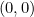and has radius. Every other circle is centred on the South pole of the previous circle and has radius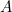times that of the previous circle. (In the first example in the main article we have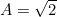, but we give a sketch of the proof for general.)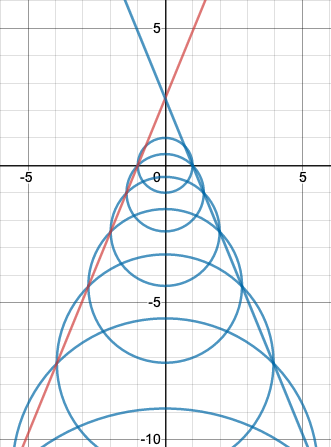The family of circles with the two lines of intersection. The y-intercept is the silver ratio.

We want to show that all the intersection points that lie on the same side of the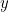-axis lie on a straight line, as shown in the diagram above.

We will also show that the two lines of intersection meet the-axis in the point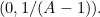If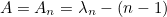we have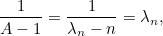as claimed in the main article.

First notice that the radius of the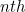circle is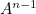. This is because the first circle has radiusand any other circle has radiustimes the radius of the previous circle.

Also notice that for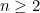thecircle has centre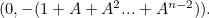That’s because the centre of the first circle isand the-coordinate of the centre of any other circle is the-coordinate of the centre of the previous circle minus the radius of the previous circle. (The first circle has centre.)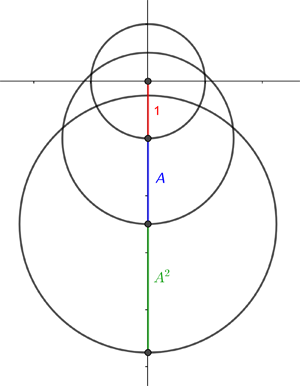The centres and radii of the first three circles.

Our proof rests on the fact that there is quite a simple transformation of the plane which sends each circle in the family to the next one along. This transformation, call it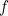, consists of a scaling by a factor of, followed by a translation by 1 in the direction of the negative-axis. In other words,sends a point with coordinates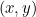to the point with coordinates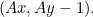We leave the following two facts for you to prove:

1. The transformationsends a circle with centre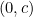and radius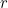to circle with centre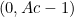and radius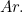2. The transformationsends a straight line with equation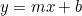to the straight line with equation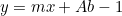.

By point (1), thecircle in our family gets sent byto a circle with radius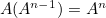and centre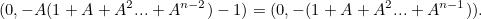And that’s exactly the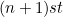circle in our family, as required.

To show that the intersection points of circles lie on a straight line as claimed, let’s first think about the line segment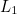which connects the intersection points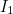and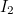as shown below.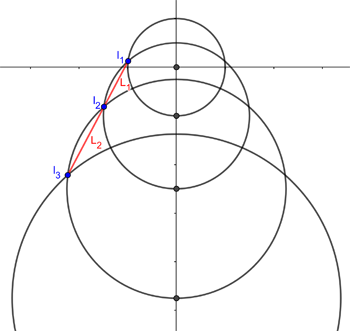Points of intersection and the line segments connecting them.

By point (2) above,sendsto another straight line segment (rather than some curvy shape). Becausesends each circle to the next one along, that line segment, call it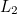is the one connecting the intersection pointsand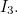Point (2) also says thatandhave the same slope — and from this it follows that,and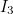all lie on a straight line. You can apply the same argument to other line segments connecting consecutive intersection points, to show that all intersection points lie on the same straight line, call it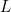.

The argument above also shows thatsendsto itself. This implies that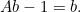Rearranging gives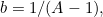as required.

Back to the main article.